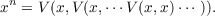#### Vol. 41, No. 2, 1972

 Download this articleFor screen For printingRecent Issues Vol. 325: 1 Vol. 324: 1  2 Vol. 323: 1  2 Vol. 322: 1  2 Vol. 321: 1  2 Vol. 320: 1  2 Vol. 319: 1  2 Vol. 318: 1  2Online Archive Volume: Issue:The Journal Subscriptions Editorial Board Officers Contacts Submission Guidelines Submission Form Policies for Authors ISSN: 1945-5844 (e-only) ISSN: 0030-8730 (print) Special Issues Author Index To Appear Other MSP Journals
Differentiable power-associative groupoids

### John P. Holmes

Vol. 41 (1972), No. 2, 391–394
##### Abstract

Suppose H is a Banach space, D is an open set of H containing 0, and V is a function from D ×D to H satisfying V (0,x) = V (x,0) = x for each x in D. If n is an integer greater than 1, denote by xn the product of n x’s associated as follows whenever the product exists.Define x0 = 0 and x1 = x.V is said to be power associative if and only if V (xn,xm) = xn+m whenever each of n and m is a nonnegative integer and xn+m exists.

Theorem A. If H and V are as above, V is power associative and continuously differentiable in the sense of Frechet on D × D then there are positive numbers a and c such that if x is in H and x< a there is a unique continuous function Tx from [0,1] to the ball of radius c centered at 0 satisfying V (Tx(s),Tx(t)) = Tx(s + t) whenever each of s,t, and s + t is in [0,1],Tx(0) = 0, and Tx(1) = x.

Primary: 46N05
Secondary: 20N99
##### Milestones